# Examples of Solutions to Problems Involving Motion of Rigid Bodies (Part - 1) Civil Engineering (CE) Notes | EduRev

## Civil Engineering (CE) : Examples of Solutions to Problems Involving Motion of Rigid Bodies (Part - 1) Civil Engineering (CE) Notes | EduRev

The document Examples of Solutions to Problems Involving Motion of Rigid Bodies (Part - 1) Civil Engineering (CE) Notes | EduRev is a part of the Civil Engineering (CE) Course Introduction to Dynamics and Vibrations- Notes, Videos, MCQs.
All you need of Civil Engineering (CE) at this link: Civil Engineering (CE)

6.7 Examples of solutions to problems involving motion of rigid bodies

The best way to learn how to use the equations in section 6.6 is just to work through a series of examples.

6.7.1 Solutions to 2D problems

Example 1: A solid of revolution (eg a cylinder or sphere) with mass M and mass moment of inertia about its COM IGzz is released from rest at the top of a ramp.  It rolls without slip. Calculate its velocity at the bottom of the ramp.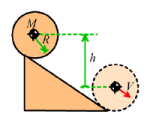• The system is conservative, so we can solve the problem using energy conservation. The energy equation tells us that the sum of kinetic and potential energy of the cylinder is constant: T0 + V0 = T+V1
• We can take the datum for potential energy to be the position of the COM at the bottom of the ramp. The initial potential energy is therefore V0 = Mgh ; the final potential energy is zero.
• The initial kinetic energy is zero, because the cylinder is stationary.   The final kinetic energy is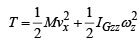• The energy equation gives  T0+V0 = T+ V1 ⇒Mgh = 1/2 Mvx2 + 1/2 IGzzωz2
• Finally, since the cylinder rolls without slip, we know that vx = -Rωz.

Hence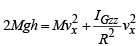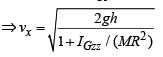This formula predicts that an object with a smaller inertia IGzz will move faster than an object with a large inertia.   A sphere rolls down the ramp more quickly than a cylinder, for example, and a solid cylinder rolls more quickly than a ring.

Example 2: For the problem treated in the preceding section, calculate the critical value of friction coefficient necessary to prevent slip at the contact.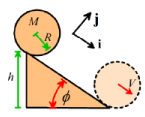If we want to learn about forces, we have to use the linear and angular momentum equations. This problem can be solved with the 2D formulas in terms of accelerations: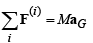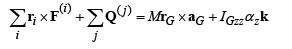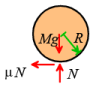• The figure shows a free body diagram for the cylinder (or sphere)
• We know that the COM is always a constant height above the ramp, so the acceleration must be parallel to i. The linear momentum equation gives(Mg sinφ − T )i + ( N− Mg cosφ ) j = MaGxi
• We can use the angular momentum equation – it is convenient to take moments about the contact point C.   (There are no torques in this problem).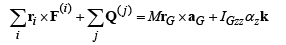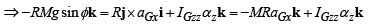• Finally, we can use the rolling wheel formula for accelerations aGx = −Rαz .
• The preceding results give: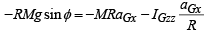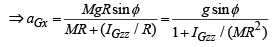• Finally, substituting back into the i components of (1):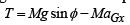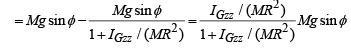• The j component of (1) gives N = Mg cosφ
• For no slip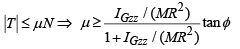The formula shows that objects with large values of IGzz / MR2 are more likely to slip. If the inertia is very small, slip will never occur. A ring will slip on a lower slope than a cylinder, which will slip on a lower slope than a sphere.

Example 3: A vertical mast can be idealized as a slender rod with length L and mass M, which is held in an inverted position by a torsional spring with stiffness κ at its base.   Find the equation of motion for the angle θ in the figure, and hence determine the natural frequency of vibration of the mast.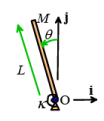This is a conservative system.  Also, the mast rotates about a fixed point.   We can analyze the problem using energy methods, and use the special formulas for rotation about a fixed point.

• The kinetic energy formula for planar motion is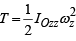• For planar motion we know that ωz =dθ/dt
• We can use the parallel axis theorem to calculate the mass moment of inertia of a rod about one end: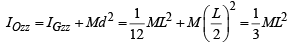• Gravity and the torsional spring both contribute to the total potential energy of the system.  The total potential energy is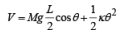• Energy conservation means that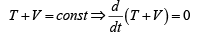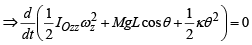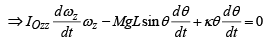• Recall that ωz = dθ/dt so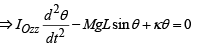• We assume that θ is small enough that sinθ ≈θ , so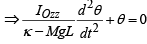• This is a standard ‘Case I’ undamped vibration EOM, so we can just read off the natural frequency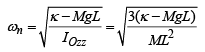Example 4: A thin uniform disk of radius R, mass m and mass moment of inertia mR2 /2 is placed on the ground with a positive velocity v0 in the horizontal direction, and a counterclockwise rotational velocity (a backspin) ω0 .  The contact between the disk and the ground has friction coefficient µ . The disk initially slips on the ground, and for a suitable range of values of ω0 and v0 its direction of motion may reverse. The goal of this problem is to calculate the conditions where this reversal will occur.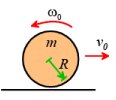Offer running on EduRev: Apply code STAYHOME200 to get INR 200 off on our premium plan EduRev Infinity!

## Introduction to Dynamics and Vibrations- Notes, Videos, MCQs

20 videos|53 docs

,

,

,

,

,

,

,

,

,

,

,

,

,

,

,

,

,

,

,

,

,

;